## Operations on Fractions

### Learning Outcomes

• Simplify fractions.
• Multiply fractions.
• Divide fractions.

Math students and working adults often find their knowledge of how to add, subtract, multiply, and divide fractions has grown rusty with disuse.  We tend to rely on calculators to do a lot of the work of fractions for us. College Algebra, though, builds up some important techniques for handling expressions and equations that are based on operations on fractions. So it is important to refamiliarize yourself with these skills. This section will remind you how to do operations on fractions. As you work through the rest of the course, you can return this section as needed for a quick reminder of operations on fractions.

Before we get started, let’s define some terminology.

• product: the result of  multiplication
• factor: something being multiplied – for  $3 \cdot 2 = 6$ , both $3$ and $2$ are factors of $6$
• numerator: the top part of a fraction – the numerator in the fraction $\Large\frac{2}{3}$ is $2$
• denominator: the bottom part of a fraction – the denominator in the fraction $\Large\frac{2}{3}$ is $3$

Certain words are used in math textbooks and by teachers to provide students with instructions on what to do with a given problem. For example, you may see instructions such as find or simplify. It is important to understand what these words mean so you can successfully work through the problems in this course. Here is a short list of some problem instructions along with their descriptions as they will be used in this module.

Instruction Interpretation
Find Perform the indicated mathematical operations which may include addition, subtraction, multiplication, division (later, use of the word find will be expanded to solving equations, as in find the value of variable).
Simplify 1) Perform the indicated mathematical operations including addition, subtraction, multiplication, division

2) Write a mathematical statement in smallest terms so there are no other mathematical operations that can be performed—often found in problems related to fractions and the order of operations

Evaluate 1) Perform the indicated mathematical operations including addition, subtraction, multiplication, division

2) Substitute a given value for a variable in an expression and then perform the indicated mathematical operations

Reduce Write a mathematical statement in smallest or lowest terms so there are no other mathematical operations that can be performed—often found in problems related to fractions or division

When you need to add or subtract fractions, you will need to first make sure that the fractions have the same denominator. The denominator tells you how many pieces the whole has been broken into, and the numerator tells you how many of those pieces you are using.

The “parts of a whole” concept can be modeled with pizzas and pizza slices. For example, imagine a pizza is cut into $4$ pieces, and someone takes $1$ piece. Now, $\Large\frac{1}{4}$ of the pizza is gone and $\Large\frac{3}{4}$ remains. Note that both of these fractions have a denominator of $4$, which refers to the number of slices the whole pizza has been cut into. What if you have another pizza that had been cut into $8$ equal parts and $3$ of those parts were gone, leaving $\Large\frac{5}{8}$?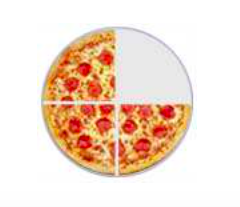How can you describe the total amount of pizza that is left with one number rather than two different fractions? You need a common denominator, technically called the least common multiple. Remember that if a number is a multiple of another, you can divide them and have no remainder.

One way to find the least common multiple of two or more numbers is to first multiply each by $1, 2, 3, 4$, etc.  For example, find the least common multiple of $2$ and $5$.

 First, list all the multiples of $2$: Then list all the multiples of 5: $2\cdot 1 = 2$ $5\cdot 1 = 5$ $2\cdot 2 = 4$ $5\cdot 2 = 10$ $2\cdot 3 = 6$ $5\cdot 3 = 15$ $2\cdot 4 = 8$ $5\cdot 4 = 20$ $2\cdot 5 = 10$ $5\cdot 5 = 25$

The smallest multiple they have in common will be the common denominator to use to rewrite each fraction into equivalent fractions. See the example below for a demonstration of our pizza problem.

### Example

One pizza, cut into four slices, has one missing. Another pizza of the same size has been cut into eight pieces, of which three have been removed. Describe the total amount of pizza left in the two pizzas using common terms.

To add fractions with unlike denominators, first rewrite them with like denominators. Then add or subtract the numerators over the common denominator.

### Adding Fractions with Unlike Denominators

1. Find a common denominator.
2. Rewrite each fraction as an equivalent fraction using the common denominator.
3. Now that the fractions have a common denominator, you can add the numerators.
4. Simplify by canceling out all common factors in the numerator and denominator.

## Simplify a Fraction

It is a common convention in mathematics to present fractions in lowest terms. We call this practice simplifying or reducing the fraction, and it can be accomplished by canceling (dividing) the common factors in a fraction’s numerator and denominator.  We can do this because a fraction represents division, and for any number $a$, $\dfrac{a}{a}=1$.

For example, to simplify $\dfrac{6}{9}$ you can rewrite $6$and $9$ using the smallest factors possible as follows:

$\dfrac{6}{9}=\dfrac{2\cdot3}{3\cdot3}$

Since there is a $3$ in both the numerator and denominator, and fractions can be considered division, we can divide the $3$ in the top by the $3$ in the bottom to reduce to $1$.

$\dfrac{6}{9}=\dfrac{2\cdot\cancel{3}}{3\cdot\cancel{3}}=\dfrac{2\cdot1}{3}=\dfrac{2}{3}$

In the next example you are shown how to add two fractions with different denominators, then simplify the answer.

### Example

Add $\Large\frac{2}{3}+\Large\frac{1}{5}$. Simplify the answer.

You can find a common denominator by finding the common multiples of the denominators. The least common multiple is the easiest to use.

### Example

Add $\Large\frac{3}{7}+\Large\frac{2}{21}$. Simplify the answer.

In the following video you will see an example of how to add two fractions with different denominators.

You can also add more than two fractions as long as you first find a common denominator for all of them. An example of a sum of three fractions is shown below. In this example, you will use the prime factorization method to find the LCM.

Add $\Large\frac{3}{4}+\Large\frac{1}{6}+\Large\frac{5}{8}$.  Simplify the answer and write as a mixed number.

What makes this example different than the previous ones? Use the box below to write down a few thoughts about how you would add three fractions with different denominators together.

## Subtract Fractions

Subtracting fractions follows the same technique as adding them. First, determine whether or not the denominators are alike. If not, rewrite each fraction as an equivalent fraction, all having the same denominator.  Below are some examples of subtracting fractions whose denominators are not alike.

### Example

Subtract $\Large\frac{1}{5}-\Large\frac{1}{6}$. Simplify the answer.

The example below shows how to use multiples to find the least common multiple, which will be the least common denominator.

### Example

Subtract $\Large\frac{5}{6}-\Large\frac{1}{4}$. Simplify the answer.

In the following video you will see an example of how to subtract fractions with unlike denominators.

## Multiply Fractions

Just as you add, subtract, multiply, and divide when working with whole numbers, you also use these operations when working with fractions.   There are many times when it is necessary to multiply fractions. A model may help you understand multiplication of fractions.

When you multiply a fraction by a fraction, you are finding a “fraction of a fraction.” Suppose you have $\Large\frac{3}{4}$ of a candy bar and you want to find $\Large\frac{1}{2}$ of the $\Large\frac{3}{4}$: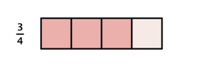By dividing each fourth in half, you can divide the candy bar into eighths.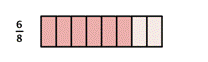Then, choose half of those to get $\Large\frac{3}{8}$.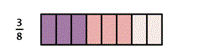In both of the above cases, to find the answer, you can multiply the numerators together and the denominators together.

### Multiplying Two Fractions

$\Large\frac{a}{b}\cdot\Large\frac{c}{d}=\Large\frac{a\cdot c}{b\cdot d}=\Large\frac{\text{product of the numerators}}{\text{product of the denominators}}$

### Multiplying More Than Two Fractions

$\Large\frac{a}{b}\cdot\Large\frac{c}{d}\cdot\Large\frac{e}{f}=\Large\frac{a\cdot c\cdot e}{b\cdot d\cdot f}$

### Example

Multiply $\Large\frac{2}{3}\cdot\Large\frac{4}{5}$

To review: if a fraction has common factors in the numerator and denominator, we can reduce the fraction to its simplified form by removing the common factors.

For example,

• Given $\Large\frac{8}{15}$, the factors of $8$ are: $1, 2, 4, 8$ and the factors of $15$ are: $1, 3, 5, 15$.  $\Large\frac{8}{15}$ is simplified because there are no common factors of $8$ and $15$.
• Given $\Large\frac{10}{15}$, the factors of $10$ are: $1, 2, 5, 10$ and the factors of $15$ are: $1, 3, 5, 15$. $\Large\frac{10}{15}$ is not simplified because $5$ is a common factor of $10$ and $15$.

You can simplify first, before you multiply two fractions, to make your work easier. This allows you to work with smaller numbers when you multiply.

In the following video you will see an example of how to multiply two fractions, then simplify the answer.

Multiply $\Large\frac{2}{3}\cdot\Large\frac{1}{4}\cdot\Large\frac{3}{5}$. Simplify the answer.

What makes this example different than the previous ones? Use the box below to write down a few thoughts about how you would multiply three fractions together.

## Divide Fractions

There are times when you need to use division to solve a problem. For example, if painting one coat of paint on the walls of a room requires $3$ quarts of paint and you have a bucket that contains $6$ quarts of paint, how many coats of paint can you paint on the walls? You divide $6$ by $3$ for an answer of $2$ coats. There will also be times when you need to divide by a fraction. Suppose painting a closet with one coat only required $\Large\frac{1}{2}$ quart of paint. How many coats could be painted with the 6 quarts of paint? To find the answer, you need to divide $2$ by the fraction, $\Large\frac{1}{2}$.

Before we begin dividing fractions, let’s cover some important terminology.

• reciprocal: two fractions are reciprocals if their product is $1$ (Don’t worry; we will show you examples of what this means.)
• quotient: the result of division

Dividing fractions requires using the reciprocal of a number or fraction. If you multiply two numbers together and get $1$ as a result, then the two numbers are reciprocals. Here are some examples of reciprocals:

Original number Reciprocal Product
$\Large\frac{3}{4}$ $\Large\frac{4}{3}$ $\Large\frac{3}{4}\cdot\Large\frac{4}{3}=\Large\frac{3\cdot 4}{4\cdot 3}=\Large\frac{12}{12}=1$
$\Large\frac{1}{2}$ $\Large\frac{2}{1}$ $\Large\frac{1}{2}\cdot\Large\frac{2}{1}=\Large\frac{1\cdot2}{2\cdot1}=\Large\frac{2}{2}=1$
$3=\Large\frac{3}{1}$ $\Large\frac{1}{3}$ $\Large\frac{3}{1}\cdot\Large\frac{1}{3}=\Large\frac{3\cdot 1}{1\cdot 3}=\Large\frac{3}{3}=1$
$2\Large\frac{1}{3}=\Large\frac{7}{3}$ $\Large\frac{3}{7}$ $\Large\frac{7}{3}\cdot\Large\frac{3}{7}=\Large\frac{7\cdot3}{3\cdot7}=\Large\frac{21}{21}=\normalsize 1$

Sometimes we call the reciprocal the “flip” of the other number: flip $\Large\frac{2}{5}$ to get the reciprocal $\Large\frac{5}{2}$.

## Division by Zero

You know what it means to divide by $2$ or divide by $10$, but what does it mean to divide a quantity by $0$? Is this even possible? Can you divide $0$ by a number? Consider the fraction

$\Large\frac{0}{8}$

We can read it as, “zero divided by eight.” Since multiplication is the inverse of division, we could rewrite this as a multiplication problem.

$\text{?}\cdot{8}=0$.

We can infer that the unknown must be $0$ since that is the only number that will give a result of $0$ when it is multiplied by $8$.

Now let’s consider the reciprocal of $\Large\frac{0}{8}$ which would be $\Large\frac{8}{0}$. If we rewrite this as a multiplication problem, we will have

$\text{?}\cdot{0}=8$.

This doesn’t make any sense. There are no numbers that you can multiply by zero to get a result of 8. The reciprocal of $\Large\frac{8}{0}$ is undefined, and in fact, all division by zero is undefined.Caution! Division by zero is undefined and so is the reciprocal of any fraction that has a zero in the numerator. For any real number a, $\Large\frac{a}{0}$ is undefined. Additionally, the reciprocal of $\Large\frac{0}{a}$ will always be undefined.

## Divide a Fraction by a Whole Number

When you divide by a whole number, you are multiplying by the reciprocal. In the painting example where you need $3$ quarts of paint for a coat and have $6$ quarts of paint, you can find the total number of coats that can be painted by dividing $6$ by $3$, $6\div3=2$. You can also multiply $6$ by the reciprocal of $3$, which is $\Large\frac{1}{3}$, so the multiplication problem becomes

$\Large\frac{6}{1}\cdot\Large\frac{1}{3}=\Large\frac{6}{3}=\normalsize2$

### Dividing is Multiplying by the Reciprocal

For all division, you can turn the operation into multiplication by using the reciprocal. Dividing is the same as multiplying by the reciprocal.

The same idea will work when the divisor (the thing being divided) is a fraction. If you have $\Large\frac{3}{4}$ of a candy bar and need to divide it among $5$ people, each person gets $\Large\frac{1}{5}$ of the available candy:

$\Large\frac{1}{5}\normalsize\text{ of }\Large\frac{3}{4}=\Large\frac{1}{5}\cdot\Large\frac{3}{4}=\Large\frac{3}{20}$

Each person gets $\Large\frac{3}{20}$ of a whole candy bar.

If you have a recipe that needs to be divided in half, you can divide each ingredient by $2$, or you can multiply each ingredient by $\Large\frac{1}{2}$ to find the new amount.

For example, dividing by $6$ is the same as multiplying by the reciprocal of $6$, which is $\Large\frac{1}{6}$. Look at the diagram of two pizzas below.  How can you divide what is left (the red shaded region) among $6$ people fairly?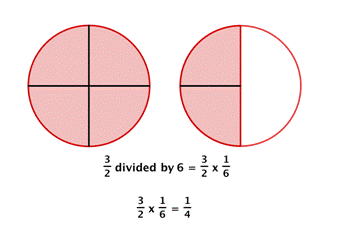Each person gets one piece, so each person gets $\Large\frac{1}{4}$ of a pizza.

Dividing a fraction by a whole number is the same as multiplying by the reciprocal, so you can always use multiplication of fractions to solve division problems.

### Example

Find $\Large\frac{2}{3}\div \normalsize 4$

### Example

Divide. $9\div\Large\frac{1}{2}$

## Divide a Fraction by a Fraction

Sometimes you need to solve a problem that requires dividing by a fraction. Suppose you have a pizza that is already cut into $4$ slices. How many $\Large\frac{1}{2}$ slices are there?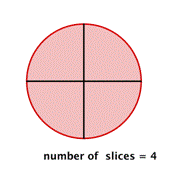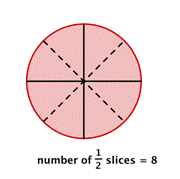There are $8$ slices. You can see that dividing $4$ by $\Large\frac{1}{2}$ gives the same result as multiplying $4$ by $2$.

What would happen if you needed to divide each slice into thirds?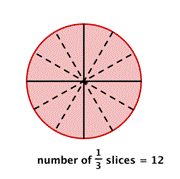You would have $12$ slices, which is the same as multiplying $4$ by $3$.

### Dividing with Fractions

1. Find the reciprocal of the number that follows the division symbol.
2. Multiply the first number (the one before the division symbol) by the reciprocal of the second number (the one after the division symbol).

Any easy way to remember how to divide fractions is the phrase “keep, change, flip.” This means to KEEP the first number, CHANGE the division sign to multiplication, and then FLIP (use the reciprocal) of the second number.

### Example

Divide $\Large\frac{2}{3}\div\Large\frac{1}{6}$

### Example

Divide $\Large\frac{3}{5}\div\Large\frac{2}{3}$

When solving a division problem by multiplying by the reciprocal, remember to write all whole numbers and mixed numbers as improper fractions. The final answer should be simplified and written as a mixed number.

In the following video you will see an example of how to divide an integer by a fraction, as well as an example of how to divide a fraction by another fraction.